# Lesson Notes By Weeks and Term - Primary 2

TERM: 2nd Term

WEEK: 5

CLASS: Primary 2

AGE: 7 years

DURATION: 5 periods of 40 minutes each

DATE:

SUBJECT: Mathematics

SPECIFIC OBJECTIVES: At the end of the lesson, the pupils should be able to

1. Solve word problems moving from pictorial representations to bar diagrams.
2. Solve subtraction word problems using bar diagrams.

INSTRUCTIONAL TECHNIQUES: Explanation, question and answer, demonstration, practical

INSTRUCTIONAL MATERIALS: bottle tops

PERIOD 1: Assessment

PRESENTATION

TEACHER’S ACTIVITY

PUPIL’S ACTIVITY

STEP 1

ORAL

ASSESSMENTS

The teacher asks questions on mental maths treated so far

Pupils respond and participate

STEP 2

DISCUSSION

The teacher discusses all the methods used by some learners in the oral assessments(some of the questions are solved on the board by the learners) and addresses any misconceptions that may have risen

Pupils pay attention and participate

STEP 3

WRITTEN ASSESSMENTS

1 Fill in the missing numbers

 T O __ 7 - 3 __ 9 2 0 2 9

O: 17 - ___ = 9

T: ___ - 30 = 20

2 Solve the following. Use the bar diagram and write a number sentence to show the answer.

I have 36 pens.18 of them are black and the rest are blue. How many blue pens do I have?3 Check this answer. Mark it with a tick or a cross.

63 – 48 = 15

4 Solve the following. Use the bar diagram and write a number sentence to show the

Pam has 25 sweets.

Rema has 16 sweets.

How many more sweets does Pam have than Rema?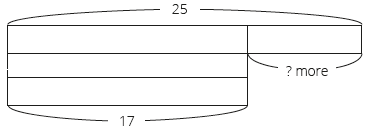Pupils attempt their class work

STEP 4

SUMMARY

The teacher marks the written assessments, corrects were necessary and commends the pupils

PERIOD 2: Word problems using bar diagrams

 PRESENTATION TEACHER’S ACTIVITY PUPIL’S ACTIVITY STEP 1MENTAL MATHS The teacher begins the lesson with some mental calculationsAdd the following numbers1 20 + 10 =    2 30 + 20 =    3 30 + 40 =    4 40 + 40 =    5 50 + 30 =   6 70 + 20 =7 50 + 50 =8 60 + 30 =9 10 + 80 =10 80 + 20 = Pupils respond and participate STEP 2CONCEPTDEVELOPMENT The teacher• Writes the following word problem on the board. This is an example of an addition(combine) problem.Nosa has 3 green marbles and2 blue marbles.How many marbles does Nosa have altogether?The word problem must be written on three lines as shown above to assist learners to identify the critical information/numbers needed to solve the problem.• Reads the problem.• Lets learners read the problem until they are able to read it fluently.• Underlines the numbers, 3 and 2.• Underlines the question (How many marbles does Nosa have altogether?) with a wavy line.• Lets learners manipulate bottle tops to represent the story.• Then, let them draw circles (with words to explain what they have drawn) in their classwork books as follows.• Draws circles on the board as above, discussing this with learners while you do it.• Asks: How can show the 2 green and 3 blue marbles in a bar diagram? (You draw boxes around each of the two groups of marbles – green and blue.)• Lets the learners draw the boxes around the circles in their classwork books and explain to each other what the relationship between the two groups is.• Lets one learner come to the board and present how she/he drew two boxes for the green and blue marbles.• Lets learners determine the operation from the bar diagram and write the number sentence that can be used to solve the problem.• Lets learners present their number sentence and confirm with the class that the correct number sentence is3 + 2 = __• Lets the learners solve the number sentence (3 + 2 = 5).• Asks: What is the answer to the word problem? (There are 5 marbles).Learners have to answer with the unit, 5 marbles. CLASS ACTIVITYThe teacher• Writes the following word problem on the board. This is an example of addition (compare).I have 4 balloons.My friend has 2 more balloons than I have.How many balloons does she have?• Uses the steps from Activity 1 to work through this problem.• Uses the diagram to illustrate using circles and create the bar diagram.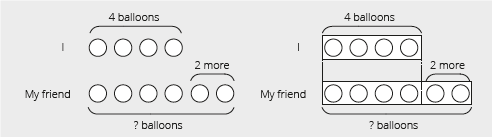• Ensures that the learners can explain the relationship between the two diagrams. (e.g. I put my balloons and my friend’s balloons into 2 different boxes.)• We draw in two rows when we compare, we draw in one row for the other type of word problem (i.e. change and combine).The number sentence is 4 + 2 = (6).The answer is 6 balloons. Pupils pay attention and participate STEP 3CLASS-WORK Learners will draw circles to represent the story in each of the problems given. Then, they should draw a bar diagram by drawing boxes around the groups of circles they drew.Finally, they should write a number sentence to show the answer to the problem. The first problem is an example of an addition (change) and the second one is an example of an addition (compare) problem.Draw circles and diagrams to help you solve these problems. Write a number sentence with the answer.a I have 5 oranges.My sister gives me 3 oranges.How many oranges do I have altogether?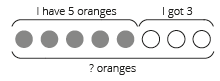b There were 6 boys in a classroom.There are 3 more girls than boys.How many girls are there?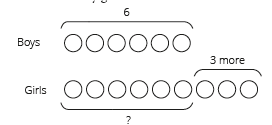Pupils attempt their class work STEP 4HOME-WORK In this homework activity, learners should draw circles to represent the story. Then, they should draw a bar diagram (boxes for each group of circles). Learners are supposed to a draw the diagram. They do not have to solve the problem. Draw circles and a bar diagram to represent this problem. I have 6 pencils.My mom buys me 2 more.How many pencils do I have altogether?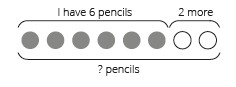The pupils writes it in their homework book STEP 5SUMMARY The teacher summarizes by reminding the pupils that in this lesson we have learnt to draw our own bar diagrams to solve addition word problems. She marks their class works, makes corrections where necessary and commends them positively

PERIOD 3: Practising bar diagrams

 PRESENTATION TEACHER’S ACTIVITY PUPIL’S ACTIVITY STEP 1MENTAL MATHS The teacher begins the lesson with some mental calculationsCalculate1 50 – 10 =    2 70 – 20 =    3 60 – 40 =    4 40 – 30 =    5 80 – 30 =    6 70 – 40 =7 90 – 50 =8 60 – 30 =9 70 – 60 =10 80 – 20 = Pupils respond and participate STEP 2CONCEPTDEVELOPMENT The teacher• Writes the following word problem on the board. This is an example ofsubtraction (change).There are 9 birds on the branch.7 of them fly away.How many birds are left?The word problem must be written on three lines as shown above to assist learners to identify the critical information/numbers needed to solve the problem.• Reads the problem.• Lets learners read the problem until they read it fluently.• Underlines the numbers, 9 and 7.• Underlines the question (How many birds are left?) with a wavy line.• Lets learners manipulate bottle tops to represent the story.• Then, let them draw circles in their classwork books (with words to explain what they have done) as follows.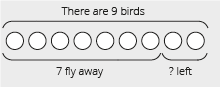• Draws circles on the board as above.• Asks: How can we show the birds that fly away and birds that remain? (You draw boxes around each of the two groups of birds – fly away and remain.)• Lets the learners draw the bar diagram/boxes around the circles in their classwork booksand explain to each other the relationship between the two.We draw in two rows when we compare, we draw in one row for the other types (i.e. change and combine).• Lets one learner come to the board and present how she/he drew two boxes for the story.• Lets learners determine the operation from the bar diagram and write the number sentence.• Lets learners present their number sentence and confirm with the class the correct number sentence.• Lets the learners solve the number sentence (9 – 7 = 2).• Asks: What is the answer for the word problem? (There are 2 birds).Learners have to answer with the unit, 2 birds. CLASS ACTIVITYThe teacher• Writes the following word problem on the board. This is an example of subtraction(compare).There are 12 sheep and8 cows in the backyard.What is the difference between the number of sheep and cows?• Uses the steps from Activity 1 to work through this problem.• Draws circles to represent the problem and then draw the bar diagram.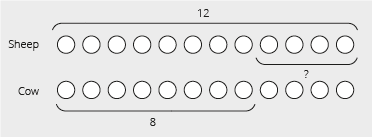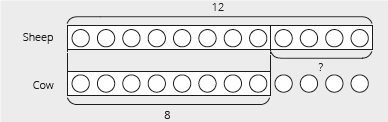Ensure that the learners can explain the relationship between the two diagrams.The number sentence is 12 – 8 = (4).The answer is: there are 4 more sheep than cows. Pupils pay attention and participate STEP 3CLASS-WORK In this activity learners must draw circles to represent the story. Then, they should draw a bar diagram (boxes for groups of circles). Finally, they should write a number sentence to show the answer. The first question is an example of subtraction (combine). The secondquestion is an example of subtraction (compare).Draw circles and diagrams to help you solve these problems. Write a number sentence with the answer. 1 There are 11 trees in the garden.6 of them are avocado trees andthe others are pawpaw trees.How many pawpaw trees are there?2 There are 13 oranges and7 apples.How many more oranges are there than apples?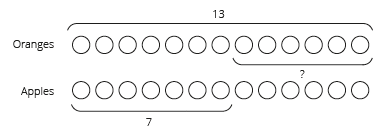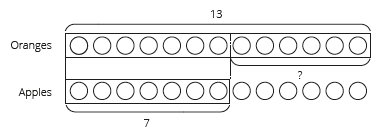Pupils attempt their class work STEP 4HOME-WORK In this homework activity, learners should draw circles to represent the story. Then, they should draw a bar diagram (boxes for each group of circles). Learners are supposed to a draw a diagram. They do not have to solve the problem. Draw circles and a bar diagram to represent the problem. There are 7 children in a playground.3 of them are boys.How many girls are there?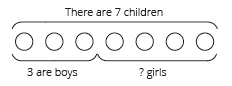The pupils writes it in their homework book STEP 5SUMMARY The teacher summarizes by reminding the pupils that in this lesson we have learnt to draw our own bar diagrams to solve subtraction word problems.She marks their class works, makes corrections where necessary and commends them positively

PERIOD 4: Solving word problems using bar diagrams

 PRESENTATION TEACHER’S ACTIVITY PUPIL’S ACTIVITY STEP 1MENTAL MATHS The teacher begins the lesson with some mental calculationsAdd the following numbers1 55 + 5 =    2 26 + 4 =    3 78 +2 =     4 95 + 5 =  5 61 + 9 =   6 99 + 1 =7 37 + 3 =8 64 + 6 =9 93 + 7 =10 72 + 8 = Pupils respond and participate STEP 2CONCEPTDEVELOPMENT The teacher• Writes the following word problem on the board. This is an example ofaddition (change).There are 6 rolls in the basket.If my father buys 2 more rolls,How many rolls are there in total? The word problem must be written on three lines as shown above to assist learners to identify the critical information/numbers needed to solve the problem.• Reads the problem.• Lets learners read the problem until they read it fluently.• Underlines the numbers, 6 and 2.• Underlines the question (How many rolls are there in total?) with a wavy line.• Lets learners manipulate bottle tops to represent the story.• Then, let them draw circles in their classwork book (with words explaining it) as follows.• Draws circles on the board as above.• Asks: How can we show the two groups of rolls – 6 rolls and 2 more rolls? (You draw boxes around each of the two groups of rolls)• Asks: How will it look if we close the lids of the boxes. (Imagine closing the boxes –you won’t see the circles anymore.)• Lets learners draw exactly the same diagram without circles in it.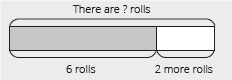This step is important for learners to understand the meaning of bar diagrams.The number sentence is 6 + 2 = (8).The answer is 8 rolls.  CLASS ACTIVITYThe teacherIn this activity learners will work in pairs to solve a word problem using a bar diagram.• Leaves Activity 1 drawn on the board.• Changes the numbers in the word problems on the board: There are 15 rolls in the basket.If my father buys 5 more rolls,How many rolls are there in total? • Asks: How do we change the diagram if the number changes? (We don’t change the diagram. We only change the numbers.)• Lets learners work in pairs and draw a bar diagram in their classwork books.Learners do not have to use bottle tops or draw circles in this activity. However, the learners who are struggling can use them.• While the learners complete this activity, walks around the class and ask learners to explain the steps to you.• Models the correct answer and how you reached it, i.e. draw the following diagram on the board to share with the whole class.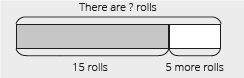The number sentence is 15 + 5 = (20).The answer is 20 rolls. Pupils pay attention and participate STEP 3CLASS-WORK The first question in this activity is an example of subtraction (change) and the second is an example of addition (combine). Learners do not need to know about this classification but itis important that they are able to do both types. Draw a bar diagram. Then, write a number sentence and the answer. a I have 5 mangoes.My brother gives me 3 more mangoes.How many mangoes do I have altogether?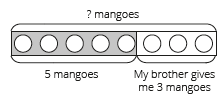b There are children in the classroom.4 of them are Grade 1s and 8 are Grade 2s.How many children are there altogether?Pupils attempt their class work STEP 4HOME-WORK Explain to learners that when they do this homework they are supposed to draw the diagram. They do not have to solve the problem.Draw a bar diagram to represent the problem. There are biscuits in a container.8 of them are choc chip and6 of them are almond.How many biscuits are there in the container? The pupils writes it in their homework book STEP 5SUMMARY The teacher summarizes by reminding the pupils that in this lesson we have learnt to draw our own bar diagrams to solve word problems.She marks their class works, makes corrections where necessary and commends them positively

PERIOD 5: Weekly Test/consolidations

TEACHER’S ACTIVITY: The teacher revises all the concepts treated from period 1-4 and gives the pupils follow through exercises, quiz and tests. She marks the exercises, makes corrections and commends the pupils positively.

PUPIL’S ACTIVITY: The pupils work on the worksheets and exercises give by the teacher individually

CONSOLIDATION

Draw a bar diagram. Then write the number sentence and solve it.

1 I have 5 apples. My friend has 3 more apples than I have. How many apples does she have?

2 There are 12 children on the playground. 6 of them leave. How many children remain?